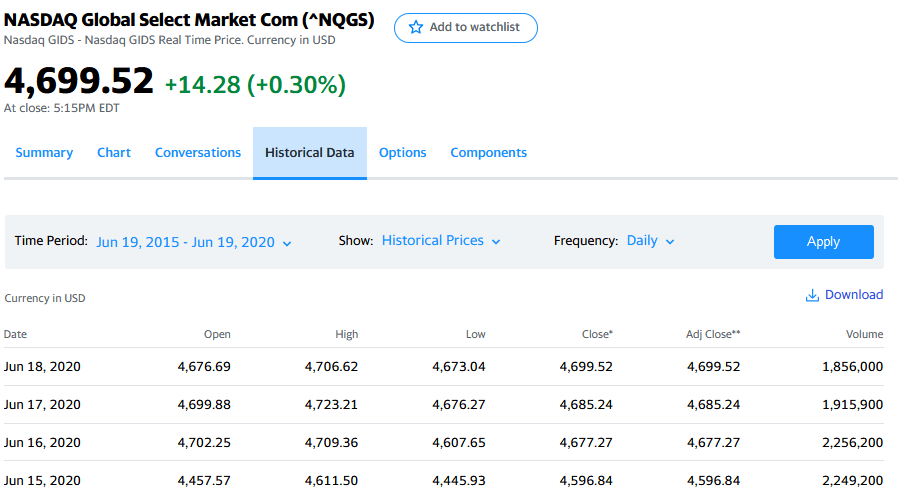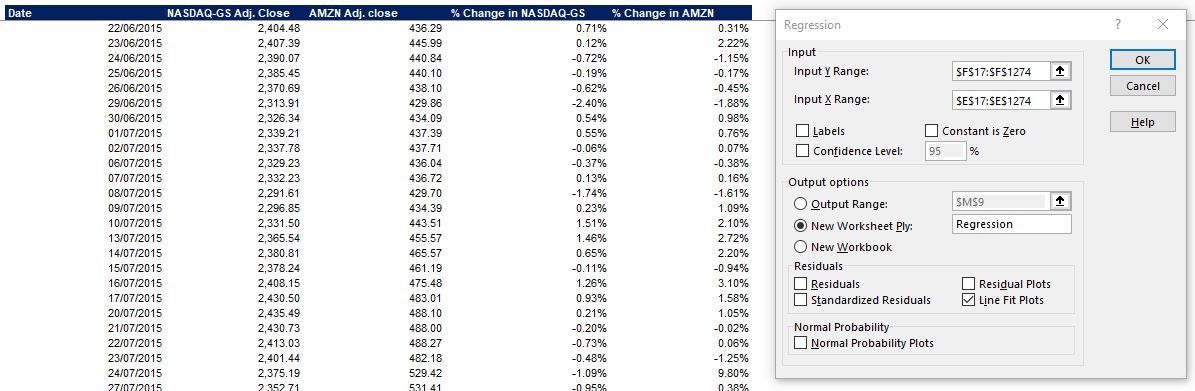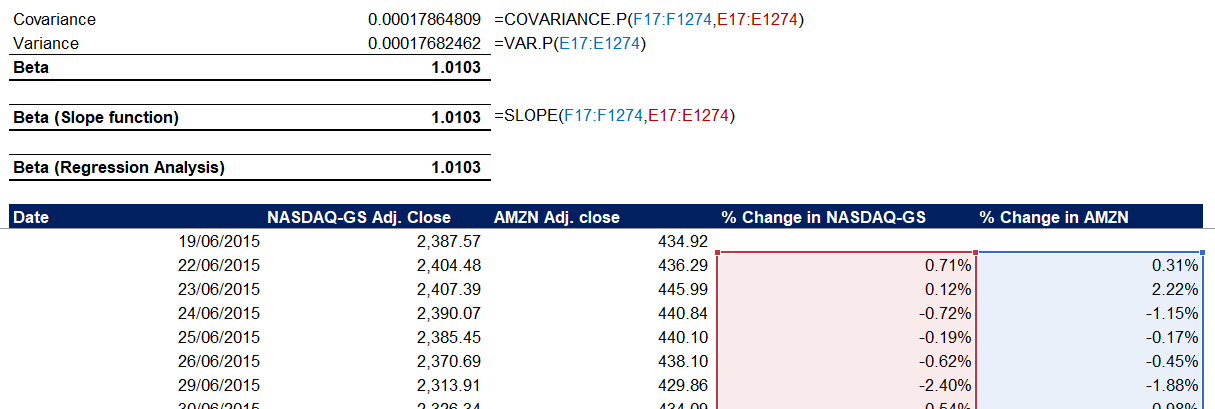## How to Calculate the Beta of a Company

Beta is a risk-reward measure from fundamental analysis to determine the volatility of an asset compared to the overall market. We consider the market to have a beta of one. Then all assets are ranked based on their deviation from the market. If an asset’s returns fluctuate more than the market, then this asset has a beta of above one and vice versa. Higher coefficient is often associated with increased risk, but it also brings the potential for higher returns.

Beta helps us identify how much risk an investor can take to gain the associated reward.

## What is the Beta Coefficient?

If we think of risk as the possibility of a value loss, we can see the beta as an appropriate measure to represent this possibility.

The beta of a company measures how its equity market value changes compared to changes in the market. We use the ratio in the Capital Asset Pricing Model (CAPM) to estimate the return of an asset.

### Systematic & Unsystematic Risk

We can look at asset-specific risk as unsystematic risk, while systematic risk represents the general market risk. If we invest in a single asset, its returns may vary wildly compared to the market returns.

As we start to build a diversified portfolio, its returns will begin to get closer to the overall market returns gradually. Diversification reduces the asset-specific unsystematic risk. The coefficient relates the systematic market risk to the unsystematic stock-specific risk by comparing the change rate between their returns.

### Capital Asset Pricing Model (CAPM) and Beta

The most popular use of the beta coefficient is to calculate the cost of equity in valuation modeling. The Capital Asset Pricing Model (CAPM) estimates beta based on systematic market risk. The method assumes a reality where investors have effectively removed unsystematic risk by diversification.

CAPM and beta give us an easy-to-calculate model that standardizes a risk measure across companies with different sizes and capital structures.

## Calculating the Coefficient

To calculate the ratio, we can look at the following formula:

Where:

• Covariance is the asset’s return relative to the market’s performance;
• The variance shows how the asset behaves in relation to the market.

The covariance is positive when one asset’s price moves up or down with another, and negative when it moves in the opposite direction.

Variance refers to the volatility of an asset’s price relative to its mean over time.

In essence, we calculate beta by multiplying the correlation of the asset’s returns and the benchmark’s performance with the standard deviation of the asset’s returns, divided over the benchmark returns’ standard deviation.

### Regression Model

We can represent beta by the slope coefficient obtained through regression analysis of the asset returns against the market returns. To calculate it, we use the following regression equation:

Where:

• ΔSi is the change in asset price;
• α is the intercept of the regression model;
• βi is the beta of the i-asset return;
• ΔM is the change in market price;
• e is the residual error.

This regression model is useful for listed companies where we have access to detailed historical data.

### Comparable Public Companies

For private companies, where no historical data is available, we can employ the comparable companies approach. The method has the following steps:

1) We identify similar companies to the business, looking at listed companies so that we can calculate or find their beta coefficients.

2) We calculate the weighted average beta and debt-to-equity (D/E) ratio for the selection of listed comparable companies.

3) In this step, we un-lever the beta coefficient using the debt-to-equity ratio. We achieve this with the following formula:

Where T is the tax rate, and D/E is the debt-to-equity ratio.

4) We then re-lever the beta with the company’s target D/E ratio.The Comparable Companies approach has one major flaw. It doesn’t account for the size difference between listed and private companies, which is usually significant.

### Earnings Beta

Large listed companies usually operate in multiple industries. This can make it hard to find appropriately comparable businesses to a private company that only runs within a single sector.

When it is too difficult to obtain reliable, comparable data, we can use the earnings beta as a proxy for the levered beta. We achieve this with historical data on changes in earnings and the market returns. Investors usually use a market index within the same region or industry, e.g., we will generally use S&P500 for US-based companies.

This method adjusts the coefficient to account for the fact that the ratio tends to gravitate towards one in the long-term. We smooth it to make it applicable to the company’s future.

We can derive the smoothing factor (α) through historical statistical analysis, but the calculations this involves are quite complex. Therefore, analysts generally use 0.33 (1/3) as a rule of thumb.

The main issue here is that private companies rarely have enough historical data for reliable regression analysis of the historical beta. Also, accounting earnings are influenced by accounting policy changes, so they might need complex adjustments to be reliable in a regression analysis.

## Analyzing the Beta Coefficient

Once we calculate the beta coefficient, we can compare it to the market or a benchmark. Based on the measure’s value, we can identify different signals.

We have the following positions for beta:

• A high beta (above 1.0) – the asset is correlated to the market or benchmark index but is more volatile and has higher potential returns;
• A coefficient around 1.0 – the asset is highly correlated to the market. This means the asset is performing in line with the industry, and these are generally the stocks investors look for;
• Low beta (above zero and below 1.0) – less volatile than the market and brings less risk;
• Beta around zero – the stock is not very correlated to the market or benchmark index;
• Negative beta (below zero) – the asset has a negative correlation to the market. In theory, this is a possibility, but it’s scarce to find an asset with a negative beta. Stocks with a coefficient below -1.0 are usually less risky but are also likely to provide lower returns. We can use these as a diversification strategy, as they can smooth the overall volatility of our portfolio.

## Example Calculation

Let’s look at how we can calculate the beta of a public entity. We will look at Amazon.com (AMZN). First, download historical data on the stock price from Yahoo Finance. I will be using the Daily data for the last five years.

We can see (below the name of the company) that the index is NasdaqGS. A quick google search shows us that NasdaqGS stands for NASDAQ Global Select Market.

We head over to the index on Yahoo Finance and download the same historical data – five years on a daily frequency.The next step is to place the Adjusted Close prices of both the AMZN stock and NASDAQ-GS index in the same table and calculate their change percentage.

From there, we can use three formulas to calculate the beta measure. First, we can calculate the covariance of the asset performance to the index performance, as well as the variance within the asset performance. Dividing one over the other gives us the beta of the Amazon.com stock.Another way is to use the SLOPE function in Excel. The first array will be the percentage change in AMZN’s stock prices, and the second will be the changes in the NASDAQ-GS index.Our third option will be to use the included Regression Analysis (Data Tab -> Analyze -> Data Analysis -> Regression). Keep in mind you need to enable the Analysis ToolPak add-in in Excel to have access to Data Analysis. We select the changes in the stock for our Y Range, and the index performance changes for our X Range.After we run the regression analysis, we get some statistical measures in a new worksheet. If we look at the coefficients column, we will find our beta ratio.The regression analysis has the option to plot a prediction for the Y values, effectively showing us the beta visually (we need to place a checkmark on the Line Fit Plots option when running the analysis).You can use any of the methods and get the same result. I usually use at least two, so I can ensure that my calculations are error-free.As we discussed, Amazon’s beta of 1.01 means the stock has a strong correlation to the market and follows its movements. The coefficient also partially explains why Amazon has been a popular stock for a while now.

## Conclusion

Betas vary across industries and companies. Many high-tech firms have ratios above one, meaning they bring a higher risk for investors, but also a potential for higher returns. It is important to remember that beta as a measure is most useful with short-term risk. By calculating and comparing betas between assets, we can determine the optimal risk-reward ratio for a portfolio.

When we use the Capital Asset Pricing Model to value private companies, we can have problems because there’s no straightforward method to estimate the equity beta reliably.

Beta is one of the statistical methods that investors use to evaluate the risk a particular asset may add to their portfolio. This, in combination with investors’ knowledge of the market, can help improve their analysis and make more appropriate investing decisions.

Please show your support by sharing the article with colleagues and friends via the social buttons on the left. Also, don’t forget to download the example Excel model below.

## And Get a FREE Benchmark Analysis Template## FCCA, FMVA

Hi! I am a finance professional with 10+ years of experience in audit, controlling, reporting, financial analysis and modeling. I am excited to delve deep into specifics of various industries, where I can identify the best solutions for clients I work with.

In my spare time, I am into skiing, hiking and running. I am also active on Instagram and YouTube, where I try different ways to express my creative side.